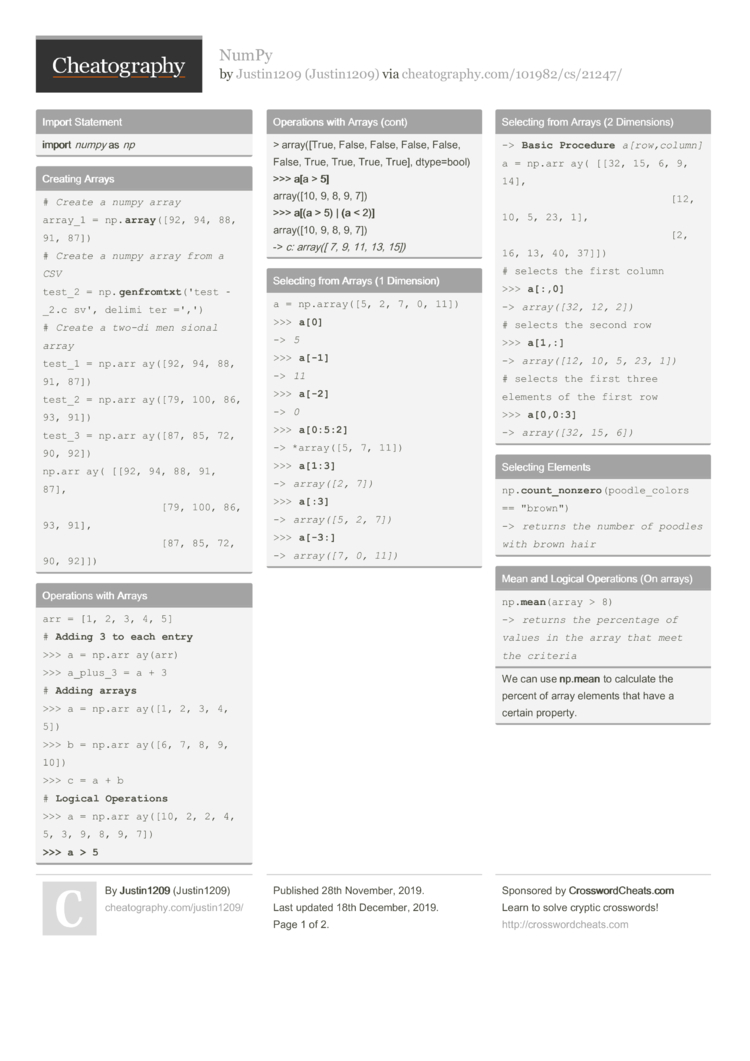# NumPy by Justin1209

### Import Statement

 import numpy as np

### Creating Arrays

 ```# Create a numpy array array_1 = np.array([92, 94, 88, 91, 87]) # Create a numpy array from a CSV test_2 = np.genfromtxt('test_2.csv', delimiter=',') # Create a two-dimensional array test_1 = np.array([92, 94, 88, 91, 87]) test_2 = np.array([79, 100, 86, 93, 91]) test_3 = np.array([87, 85, 72, 90, 92]) np.array([[92, 94, 88, 91, 87],           [79, 100, 86, 93, 91],           [87, 85, 72, 90, 92]])```

### Operations with Arrays

 ```arr = [1, 2, 3, 4, 5] # Adding 3 to each entry >>> a = np.array(arr) >>> a_plus_3 = a + 3 # Adding arrays >>> a = np.array([1, 2, 3, 4, 5]) >>> b = np.array([6, 7, 8, 9, 10]) >>> c = a + b # Logical Operations >>> a = np.array([10, 2, 2, 4, 5, 3, 9, 8, 9, 7]) >>> a > 5 array([True, False, False, False, False, False, True, True, True, True], dtype=bool) >>> a[a > 5] array([10, 9, 8, 9, 7]) >>> a[(a > 5) | (a < 2)] array([10, 9, 8, 9, 7]) -> c: array([ 7, 9, 11, 13, 15])```

### Selecting from Arrays (1 Dimension)

 ```a = np.array([5, 2, 7, 0, 11]) >>> a -> 5 >>> a[-1] -> 11 >>> a[-2] -> 0 >>> a[0:5:2] -> *array([5, 7, 11]) >>> a[1:3] -> array([2, 7]) >>> a[:3] -> array([5, 2, 7]) >>> a[-3:] -> array([7, 0, 11])```

### Selecting from Arrays (2 Dimens­ions)

 ```-> Basic Procedure a[row,column] a = np.array([[32, 15, 6, 9, 14],               [12, 10, 5, 23, 1],               [2, 16, 13, 40, 37]]) # selects the first column >>> a[:,0] -> array([32, 12, 2]) # selects the second row >>> a[1,:] -> array([12, 10, 5, 23, 1]) # selects the first three elements of the first row >>> a[0,0:3] -> array([32, 15, 6])```

### Selecting Elements

 ```np.count_nonzero(poodle_colors == "brown") -> returns the number of poodles with brown hair```

### Mean and Logical Operations (On arrays)

 ```np.mean(array > 8) -> returns the percentage of values in the array that meet the criteria```
We can use np.m­ean to calculate the percent of array elements that have a certain property.

### Mean over 2 Dimens­ional Arrays

 ```>>> ring_toss = np.array([[1, 0, 0],                           [0, 0, 1],                           [1, 0, 1]]) >>> np.mean(ring_toss) 0.44 -> Overall Average >>> np.mean(ring_toss, axis=1) array([ 0.33, 0.33, 0.67]) -> Average per row >>> np.mean(ring_toss, axis=0) array([ 0.67, 0. , 0.67]) -> Average per column```

### Dealing with Outliers

 ```# Sort the Dataset np.sort(array) -> Outliers are clearly visible now```

### Percen­tiles

 ```d = np.array([1, 2, 3, 4, 4, 4, 6, 6, 7, 8, 8]) np.percentile(d, 40) -> 4.00```

### Generate Normal Distri­bution

 ```# Generate own Normal Distribution Set -> np.random.normal(loc, scale, size) loc: the mean for the normal distribution scale: the standard deviation of the distribution size: the number of random numbers to generate```
68% of our samples will fall between +/- 1 standard deviat­ion of the mean

95% of our samples will fall between +/- 2 standard deviat­ions of the mean

99.7% of our samples will fall between +/- 3 standard deviat­ions of the mean

### Binomial Distri­bution

 ```np.random.binomial(N, P, size) N: The number of samples or trials P: The probability of success size: The number of experiments #Basketball Example  Let's generate 10,000 "experiments"  N = 10 shots  P = 0.30 (30% he'll get a free throw) -> a = np.random.binomial(10, 0.3, 10000) # Probability that he makes 4 Shots: prob = np.mean(a == 4)```
The binomial distri­but­ion can help us. It tells us how likely it is for a certain number of “succe­sses” to happen, given a probab­ility of success and a number of trials.2 Pages
//media.cheatography.com/storage/thumb/justin1209_numpy.750.jpg

PDF (recommended)